# Topic 4 Chemical Reactions Chemical reactions are the

• Slides: 74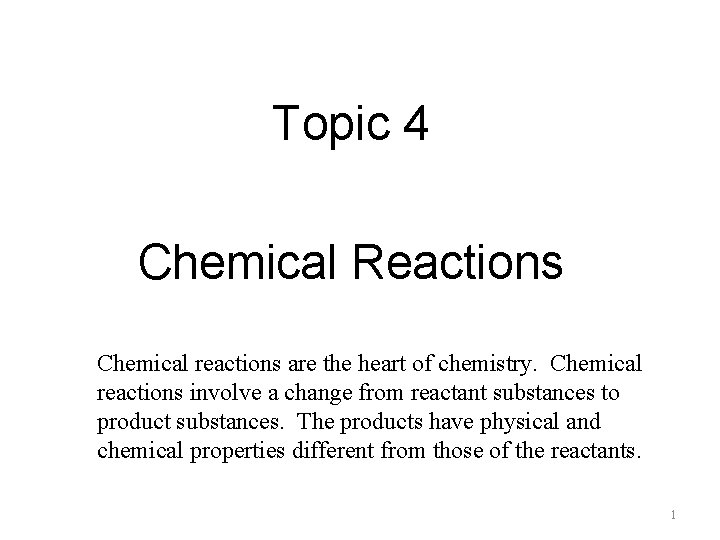Topic 4 Chemical Reactions Chemical reactions are the heart of chemistry. Chemical reactions involve a change from reactant substances to product substances. The products have physical and chemical properties different from those of the reactants. 1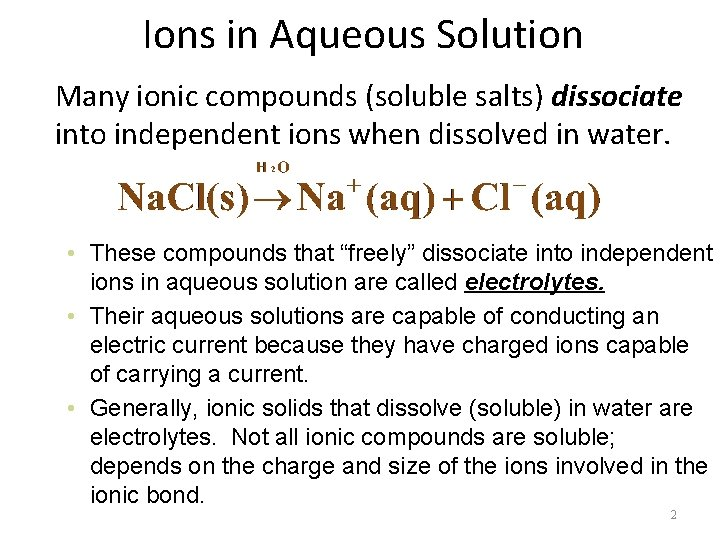Ions in Aqueous Solution Many ionic compounds (soluble salts) dissociate into independent ions when dissolved in water. • These compounds that “freely” dissociate into independent ions in aqueous solution are called electrolytes. • Their aqueous solutions are capable of conducting an electric current because they have charged ions capable of carrying a current. • Generally, ionic solids that dissolve (soluble) in water are electrolytes. Not all ionic compounds are soluble; depends on the charge and size of the ions involved in the ionic bond. 2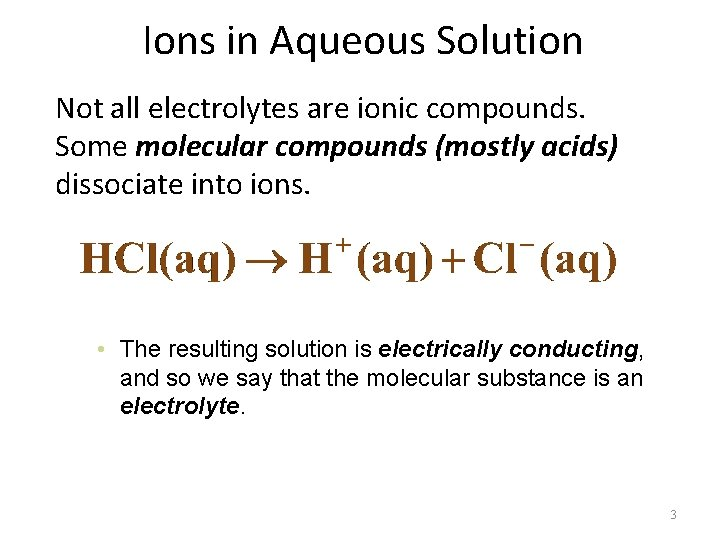Ions in Aqueous Solution Not all electrolytes are ionic compounds. Some molecular compounds (mostly acids) dissociate into ions. • The resulting solution is electrically conducting, and so we say that the molecular substance is an electrolyte. 3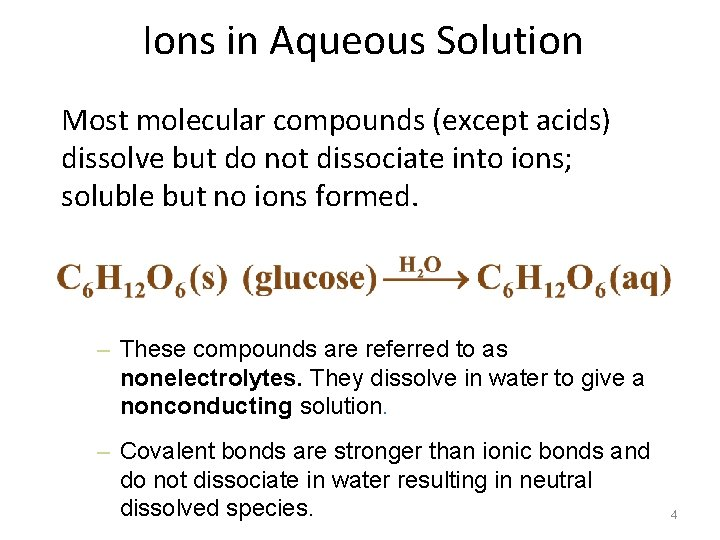Ions in Aqueous Solution Most molecular compounds (except acids) dissolve but do not dissociate into ions; soluble but no ions formed. – These compounds are referred to as nonelectrolytes. They dissolve in water to give a nonconducting solution. – Covalent bonds are stronger than ionic bonds and do not dissociate in water resulting in neutral dissolved species. 4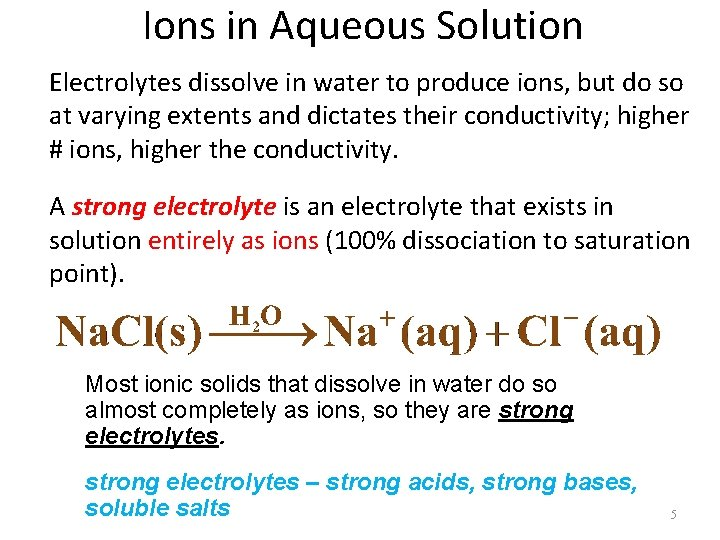Ions in Aqueous Solution Electrolytes dissolve in water to produce ions, but do so at varying extents and dictates their conductivity; higher # ions, higher the conductivity. A strong electrolyte is an electrolyte that exists in solution entirely as ions (100% dissociation to saturation point). Most ionic solids that dissolve in water do so almost completely as ions, so they are strong electrolytes – strong acids, strong bases, soluble salts 5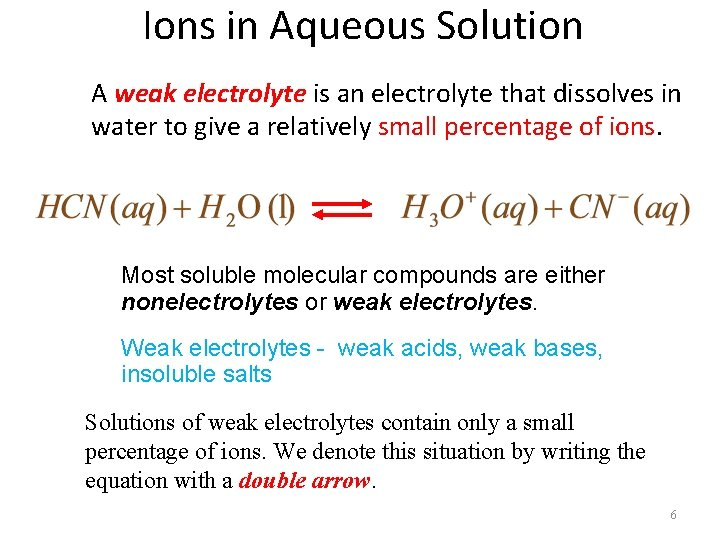Ions in Aqueous Solution A weak electrolyte is an electrolyte that dissolves in water to give a relatively small percentage of ions. Most soluble molecular compounds are either nonelectrolytes or weak electrolytes. Weak electrolytes - weak acids, weak bases, insoluble salts Solutions of weak electrolytes contain only a small percentage of ions. We denote this situation by writing the equation with a double arrow. 6We’ve stated the terms strong acid, strong base, soluble salt, insoluble salt, but we haven’t describe how to determine which species fall under these terms. To be able to write chemical reactions correctly, we will need to understand solubility and how strong and weak species dissociate in water. The first thing we will cover is the solubility rules for ionic compounds. You must know the solubility rules to distinguish between soluble and insoluble salts. Soluble salts (aq) dissociate 100% into ions up to their saturation point while insoluble salts (s) have very little dissociation. 7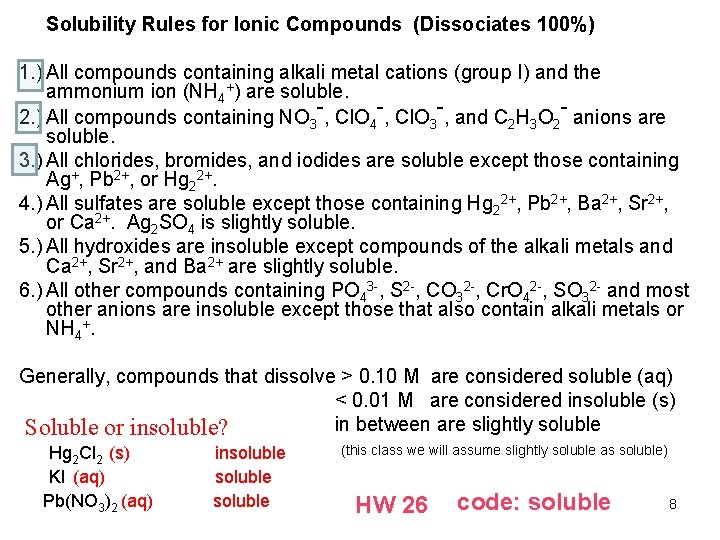Solubility Rules for Ionic Compounds (Dissociates 100%) 1. ) All compounds containing alkali metal cations (group I) and the ammonium ion (NH 4+) are soluble. 2. ) All compounds containing NO 3 -, Cl. O 4 -, Cl. O 3 -, and C 2 H 3 O 2 - anions are soluble. 3. ) All chlorides, bromides, and iodides are soluble except those containing Ag+, Pb 2+, or Hg 22+. 4. ) All sulfates are soluble except those containing Hg 22+, Pb 2+, Ba 2+, Sr 2+, or Ca 2+. Ag 2 SO 4 is slightly soluble. 5. ) All hydroxides are insoluble except compounds of the alkali metals and Ca 2+, Sr 2+, and Ba 2+ are slightly soluble. 6. ) All other compounds containing PO 43 -, S 2 -, CO 32 -, Cr. O 42 -, SO 32 - and most other anions are insoluble except those that also contain alkali metals or NH 4+. Generally, compounds that dissolve > 0. 10 M are considered soluble (aq) < 0. 01 M are considered insoluble (s) in between are slightly soluble Soluble or insoluble? Hg 2 Cl 2 (s) KI (aq) Pb(NO 3)2 (aq) insoluble (this class we will assume slightly soluble as soluble) HW 26 code: soluble 8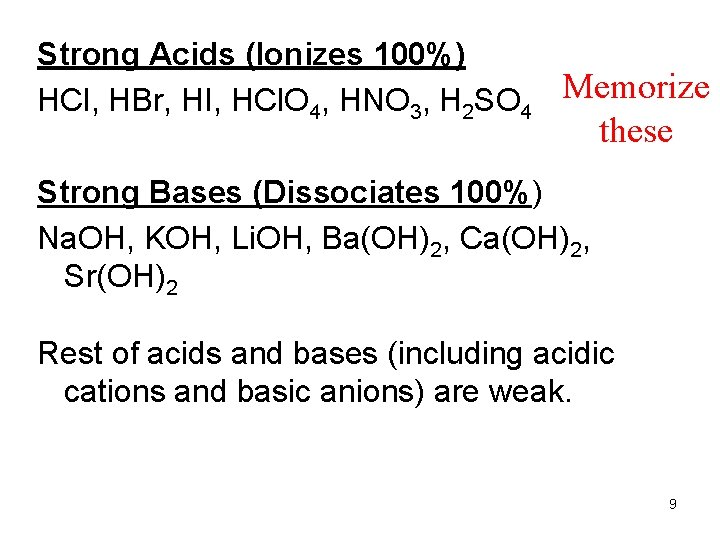Strong Acids (Ionizes 100%) HCl, HBr, HI, HCl. O 4, HNO 3, H 2 SO 4 Memorize these Strong Bases (Dissociates 100%) Na. OH, KOH, Li. OH, Ba(OH)2, Ca(OH)2, Sr(OH)2 Rest of acids and bases (including acidic cations and basic anions) are weak. 9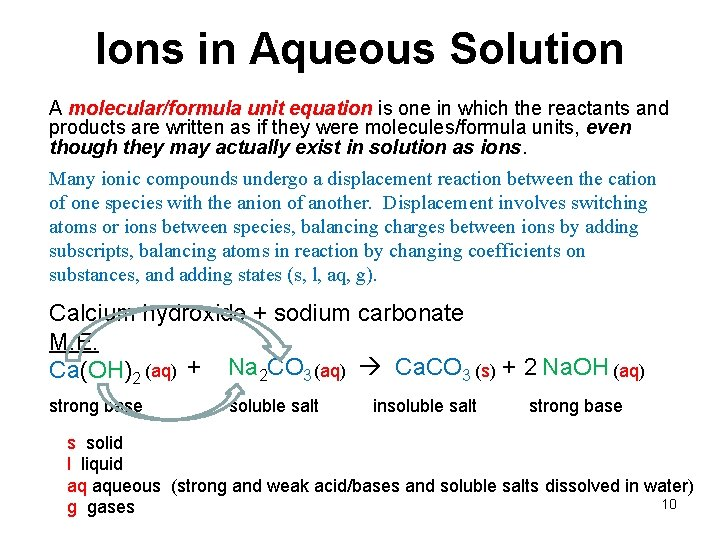Ions in Aqueous Solution A molecular/formula unit equation is one in which the reactants and products are written as if they were molecules/formula units, even though they may actually exist in solution as ions. Many ionic compounds undergo a displacement reaction between the cation of one species with the anion of another. Displacement involves switching atoms or ions between species, balancing charges between ions by adding subscripts, balancing atoms in reaction by changing coefficients on substances, and adding states (s, l, aq, g). Calcium hydroxide + sodium carbonate M. E. Ca(OH)2 (aq) + Na 2 CO 3 (aq) Ca. CO 3 (s) + 2 Na. OH (aq) strong base soluble salt insoluble salt strong base s solid l liquid aq aqueous (strong and weak acid/bases and soluble salts dissolved in water) 10 g gasesIons in Aqueous Solution An total ionic equation represents strong electrolytes as separate independent ions. This is a more accurate representation of the way electrolytes behave in solution. – A total ionic equation is a chemical equation in which strong electrolytes (such as soluble ionic compounds, strong acids/bases) are written as separate ions in solution. (note: g, l, insoluble salts (s), weak acid/bases do not break up into ions) M. E. Ca(OH)2 (aq) + strong base Na 2 CO 3 (aq) Ca. CO 3 (s) + 2 Na. OH (aq) soluble salt insoluble salt strong base Total ionic Ca 2+ (aq) + 2 OH- (aq) + 2 Na+ (aq) + CO 32 - (aq) Ca. CO 3 (s) + 2 Na+ (aq) + 2 OH- (aq) 11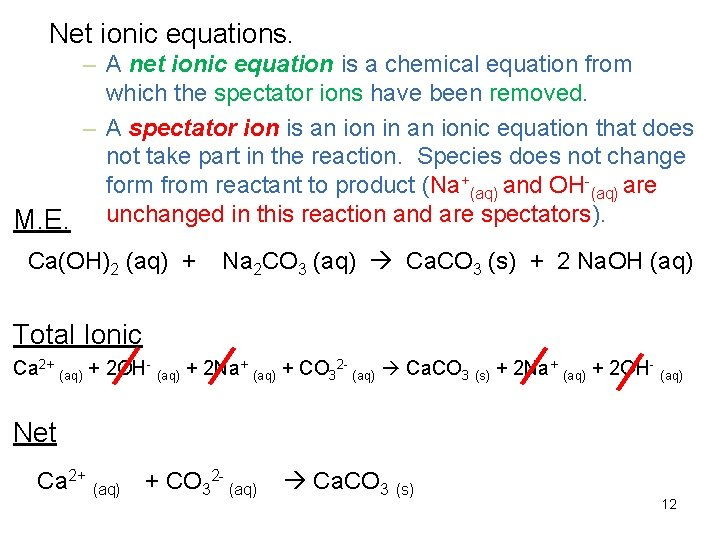Net ionic equations. – A net ionic equation is a chemical equation from which the spectator ions have been removed. – A spectator ion is an ion in an ionic equation that does not take part in the reaction. Species does not change form from reactant to product (Na+(aq) and OH-(aq) are M. E. unchanged in this reaction and are spectators). Ca(OH)2 (aq) + Na 2 CO 3 (aq) Ca. CO 3 (s) + 2 Na. OH (aq) Total Ionic Ca 2+ (aq) + 2 OH- (aq) + 2 Na+ (aq) + CO 32 - (aq) Ca. CO 3 (s) + 2 Na+ (aq) + 2 OH- (aq) Net Ca 2+ (aq) + CO 32 - (aq) Ca. CO 3 (s) 12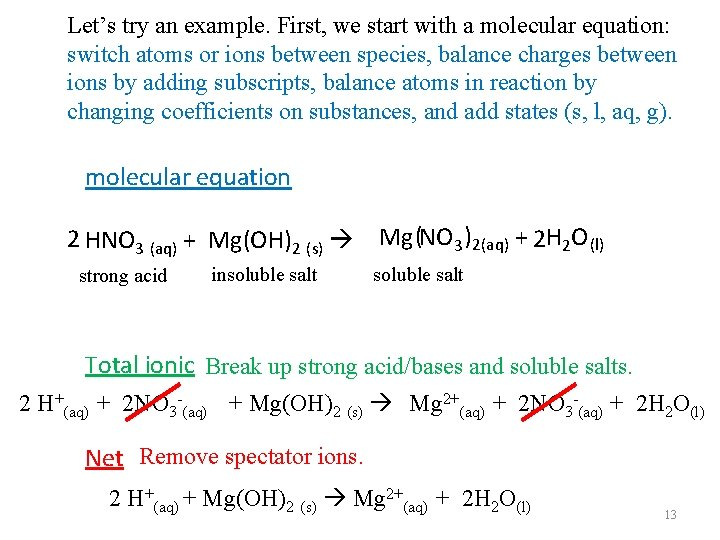Let’s try an example. First, we start with a molecular equation: switch atoms or ions between species, balance charges between ions by adding subscripts, balance atoms in reaction by changing coefficients on substances, and add states (s, l, aq, g). molecular equation 2 HNO 3 (aq) + Mg(OH)2 (s) Mg (NO 3 )2(aq) + 2 H 2 O (l) strong acid insoluble salt Total ionic Break up strong acid/bases and soluble salts. 2 H+(aq) + 2 NO 3 -(aq) + Mg(OH)2 (s) Mg 2+(aq) + 2 NO 3 -(aq) + 2 H 2 O(l) Net Remove spectator ions. 2 H+(aq) + Mg(OH)2 (s) Mg 2+(aq) + 2 H 2 O(l) 13Ions in Aqueous Solution Molecular and Ionic Equations HCN (aq) + Na. OH (aq) weak acid strong base Na. CN(aq) (l) + H 2 O soluble salt Total ionic HCN (aq) + Na+(aq) + OH-(aq) Na+(aq) + CN-(aq) + H 2 O(l) Net HCN(aq) + OH-(aq) CN-(aq) + H 2 O(l) 14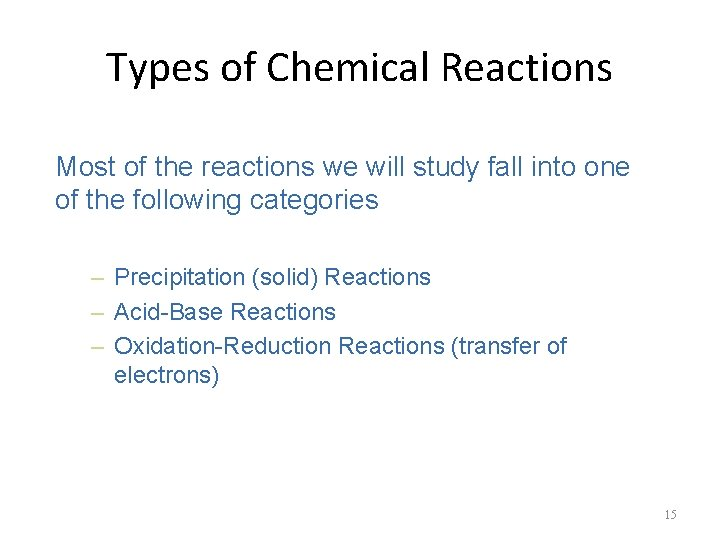Types of Chemical Reactions Most of the reactions we will study fall into one of the following categories – Precipitation (solid) Reactions – Acid-Base Reactions – Oxidation-Reduction Reactions (transfer of electrons) 15Types of Chemical Reactions Precipitation Reactions A precipitation reaction occurs in aqueous solution because one product is insoluble. – A precipitate (ppt) is an insoluble solid compound formed during a chemical reaction in solution. – For example, the reaction of sodium chloride with silver nitrate forms Ag. Cl(s), an insoluble precipitate. Is this a ppt rxn? Na. Cl (aq) + Fe(NO 3)2 (aq) Fe. Cl 2 (aq) + 2 Na. NO 3(aq) HW 27 No ppt is formed; therefore, not a ppt rxn - all aq ions; code: ppt 16 basically, no reaction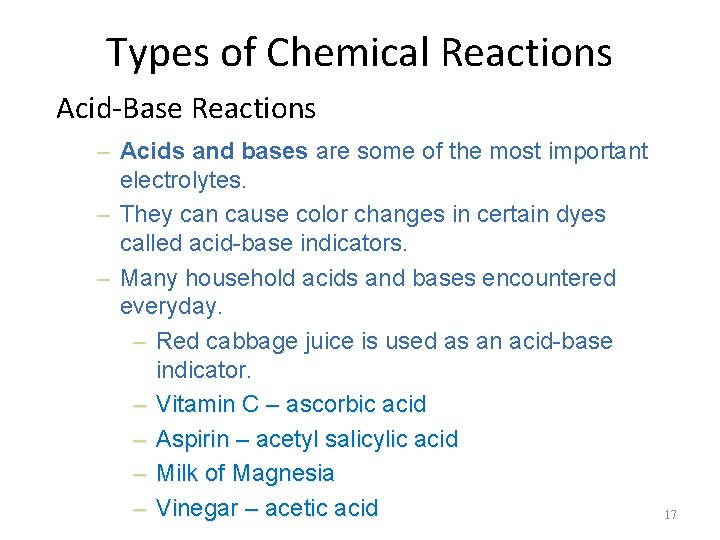Types of Chemical Reactions Acid-Base Reactions – Acids and bases are some of the most important electrolytes. – They can cause color changes in certain dyes called acid-base indicators. – Many household acids and bases encountered everyday. – Red cabbage juice is used as an acid-base indicator. – Vitamin C – ascorbic acid – Aspirin – acetyl salicylic acid – Milk of Magnesia – Vinegar – acetic acid 17Types of Chemical Reactions Different ways to define acids/bases The Arrhenius Concept – The Arrhenius concept defines acids as substances that produce hydrogen ions, H+, (also known as hydronium ions, H 3 O+) when dissolved in water. – An example is nitric acid, HNO 3, a molecular substance that dissolves in water to give H+ and NO 3 -. HNO 3(aq) + H 2 O(l) H 3 O+(aq) + NO 3 -(aq) Note: You will encounter acid ionization equations written both ways: as a dissociation and an ionization. 18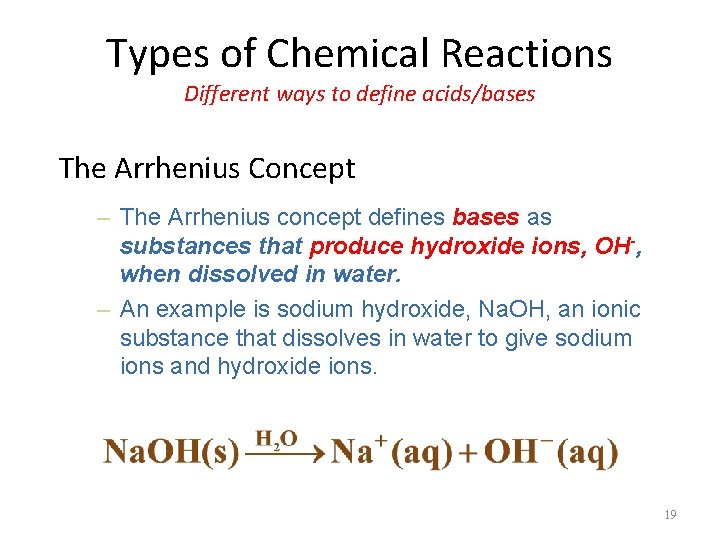Types of Chemical Reactions Different ways to define acids/bases The Arrhenius Concept – The Arrhenius concept defines bases as substances that produce hydroxide ions, OH-, when dissolved in water. – An example is sodium hydroxide, Na. OH, an ionic substance that dissolves in water to give sodium ions and hydroxide ions. 19Types of Chemical Reactions Different ways to define acids/bases By Arrhenius concept is ammonia an acid or base? By Arrhenius concept, ammonia produces OH-; therefore, it is a base. However, this concept does not cover all acids/bases that exist which gets us to a more useful acid/base concept especially when dealing with aqueous solutions. 20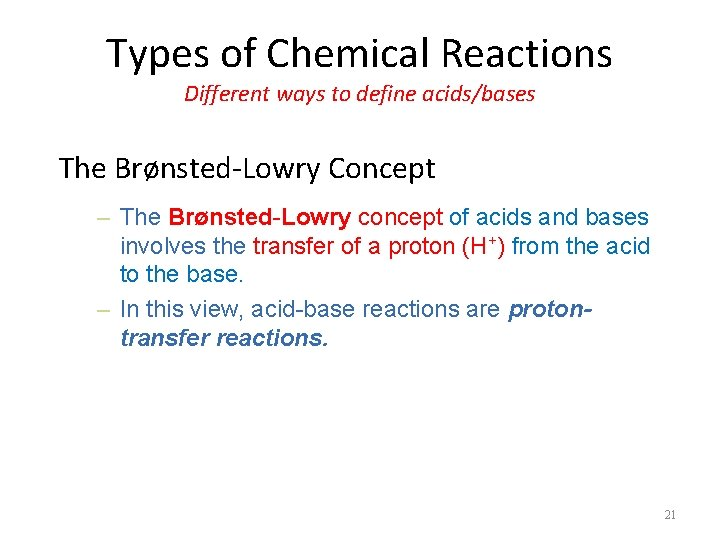Types of Chemical Reactions Different ways to define acids/bases The Brønsted-Lowry Concept – The Brønsted-Lowry concept of acids and bases involves the transfer of a proton (H+) from the acid to the base. – In this view, acid-base reactions are protontransfer reactions. 21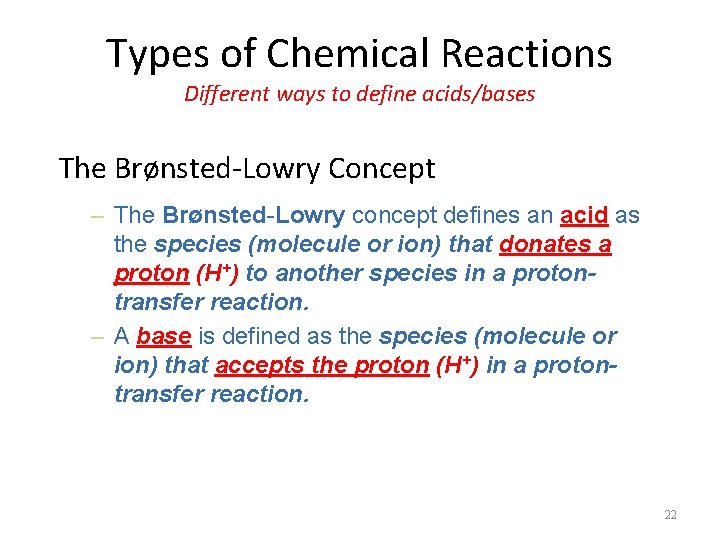Types of Chemical Reactions Different ways to define acids/bases The Brønsted-Lowry Concept – The Brønsted-Lowry concept defines an acid as the species (molecule or ion) that donates a proton (H+) to another species in a protontransfer reaction. – A base is defined as the species (molecule or ion) that accepts the proton (H+) in a protontransfer reaction. 22Types of Chemical Reactions Different ways to define acids/bases The Brønsted-Lowry Concept base acid H+ We obtained the same conclusion that NH 3 is a base using this theory; however, we didn’t look at the production of OH- to make the decision. It was based on NH 3 accepting a proton in the aqueous rxn. The H 2 O molecule is the acid because it donates a proton. The NH 3 molecule is a base, because it accepts a proton. Using Brønsted-Lowry concept makes it easy to write acid-base reactions. You remove a proton off the acid component and place it on the base component giving you the products. Water is amphiprotic meaning it can act as an acid or base. Since NH 3 is a better base, water is the acid in this 23 reaction.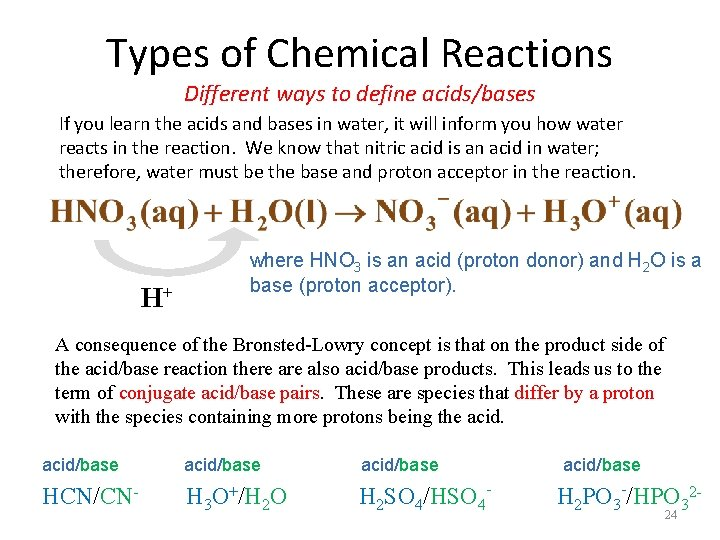Types of Chemical Reactions Different ways to define acids/bases If you learn the acids and bases in water, it will inform you how water reacts in the reaction. We know that nitric acid is an acid in water; therefore, water must be the base and proton acceptor in the reaction. H+ where HNO 3 is an acid (proton donor) and H 2 O is a base (proton acceptor). A consequence of the Bronsted-Lowry concept is that on the product side of the acid/base reaction there also acid/base products. This leads us to the term of conjugate acid/base pairs. These are species that differ by a proton with the species containing more protons being the acid/base HCN/CN- H 3 O+/H 2 O H 2 SO 4/HSO 4 - H 2 PO 3 -/HPO 3224Types of Chemical Reactions Different ways to define acids/bases In summary, – The Arrhenius concept acid: proton (H+) producer base: hydroxide ion (OH-) producer – The Brønsted-Lowry concept acid: proton (H+) donor base: proton (H+) acceptor HW 28 code: acid 25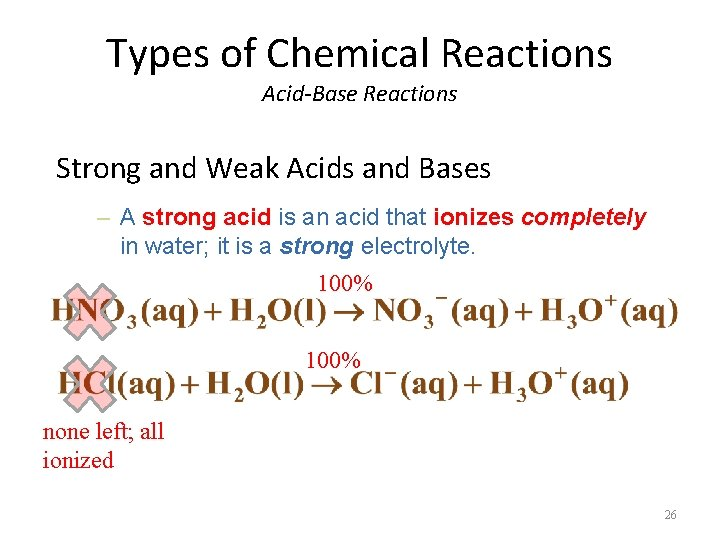Types of Chemical Reactions Acid-Base Reactions Strong and Weak Acids and Bases – A strong acid is an acid that ionizes completely in water; it is a strong electrolyte. 100% none left; all ionized 26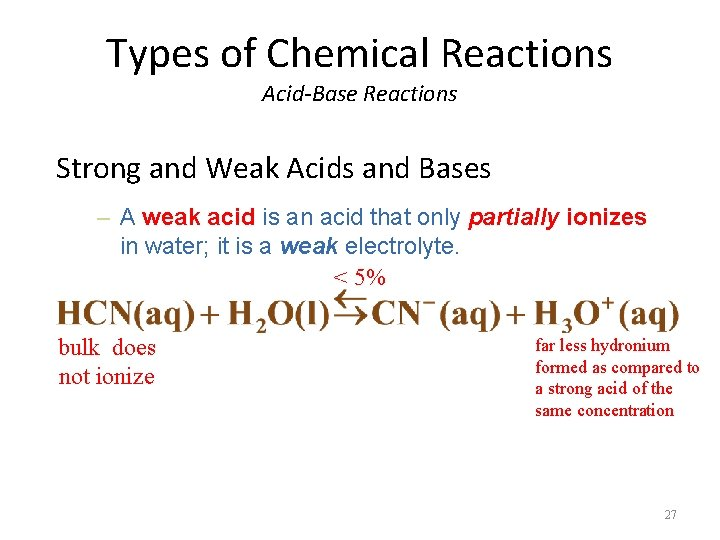Types of Chemical Reactions Acid-Base Reactions Strong and Weak Acids and Bases – A weak acid is an acid that only partially ionizes in water; it is a weak electrolyte. < 5% bulk does not ionize far less hydronium formed as compared to a strong acid of the same concentration 27Types of Chemical Reactions Acid-Base Reactions Strong and Weak Acids and Bases – A strong base is a base that is present entirely as ions, one of which is OH-; it is a strong electrolyte. 100% 28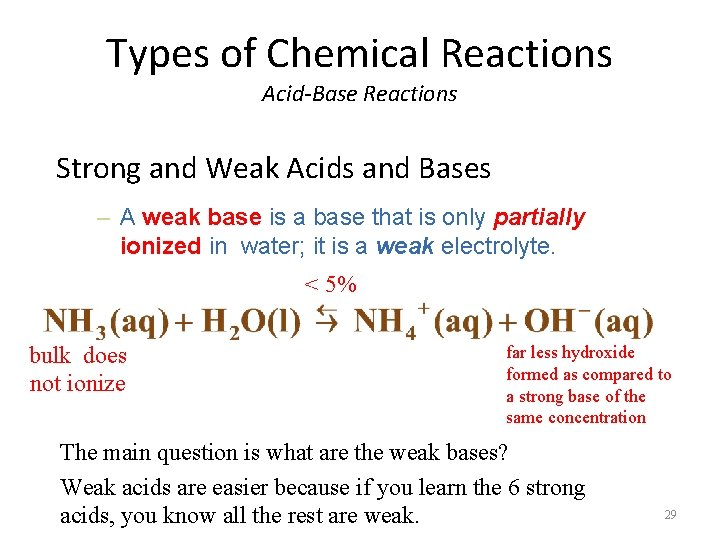Types of Chemical Reactions Acid-Base Reactions Strong and Weak Acids and Bases – A weak base is a base that is only partially ionized in water; it is a weak electrolyte. < 5% bulk does not ionize far less hydroxide formed as compared to a strong base of the same concentration The main question is what are the weak bases? Weak acids are easier because if you learn the 6 strong acids, you know all the rest are weak. 29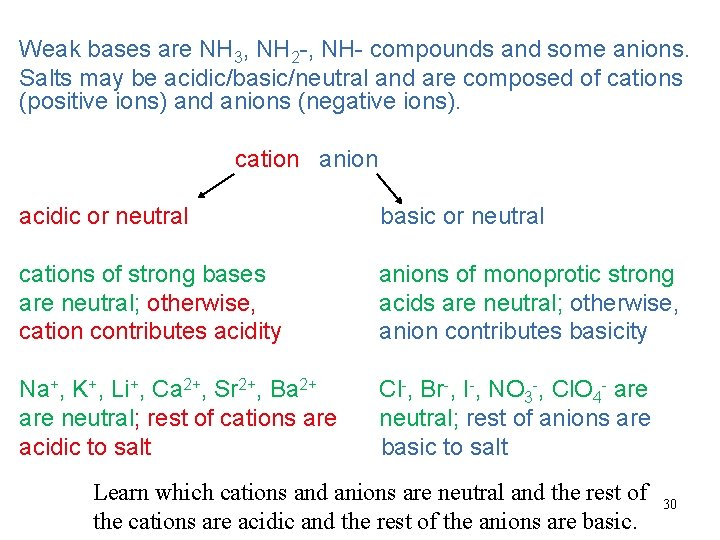Weak bases are NH 3, NH 2 -, NH- compounds and some anions. Salts may be acidic/basic/neutral and are composed of cations (positive ions) and anions (negative ions). cation anion acidic or neutral basic or neutral cations of strong bases are neutral; otherwise, cation contributes acidity anions of monoprotic strong acids are neutral; otherwise, anion contributes basicity Na+, K+, Li+, Ca 2+, Sr 2+, Ba 2+ are neutral; rest of cations are acidic to salt Cl-, Br-, I-, NO 3 -, Cl. O 4 - are neutral; rest of anions are basic to salt Learn which cations and anions are neutral and the rest of the cations are acidic and the rest of the anions are basic. 30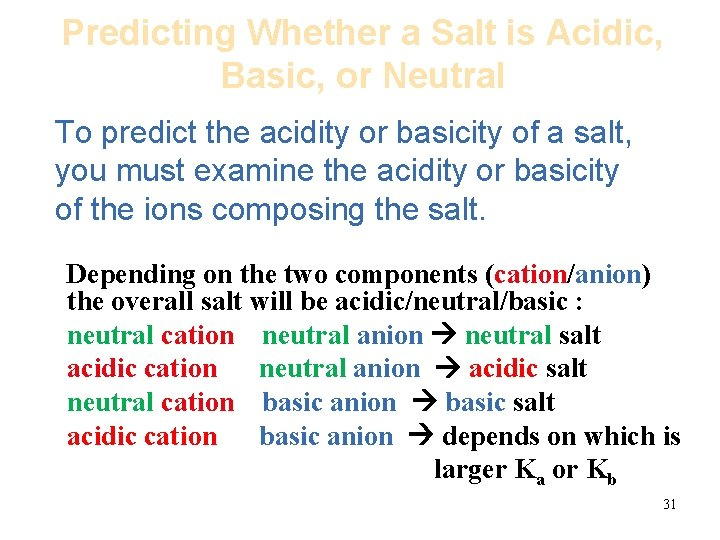Predicting Whether a Salt is Acidic, Basic, or Neutral To predict the acidity or basicity of a salt, you must examine the acidity or basicity of the ions composing the salt. Depending on the two components (cation/anion) the overall salt will be acidic/neutral/basic : neutral cation neutral anion neutral salt acidic cation neutral anion acidic salt neutral cation basic anion basic salt acidic cation basic anion depends on which is larger Ka or Kb 31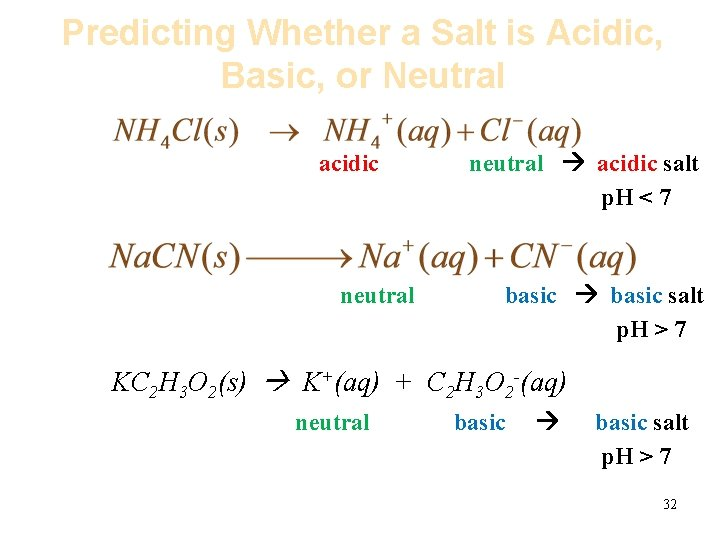Predicting Whether a Salt is Acidic, Basic, or Neutral acidic neutral acidic salt p. H < 7 basic salt p. H > 7 KC 2 H 3 O 2(s) K+(aq) + C 2 H 3 O 2 -(aq) neutral basic salt p. H > 7 32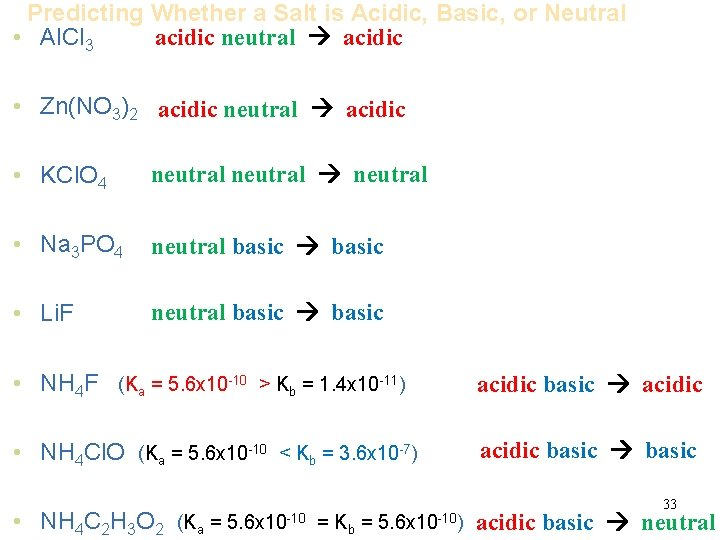Predicting Whether a Salt is Acidic, Basic, or Neutral acidic neutral acidic • Al. Cl 3 • Zn(NO 3)2 acidic neutral acidic • KCl. O 4 neutral • Na 3 PO 4 neutral basic • Li. F neutral basic • NH 4 F (Ka = 5. 6 x 10 -10 > Kb = 1. 4 x 10 -11) acidic basic acidic • NH 4 Cl. O (Ka = 5. 6 x 10 -10 < Kb = 3. 6 x 10 -7) acidic basic • NH 4 C 2 H 3 O 2 (Ka = 5. 6 x 10 -10 = Kb = 5. 6 x 10 -10) 33 acidic basic neutral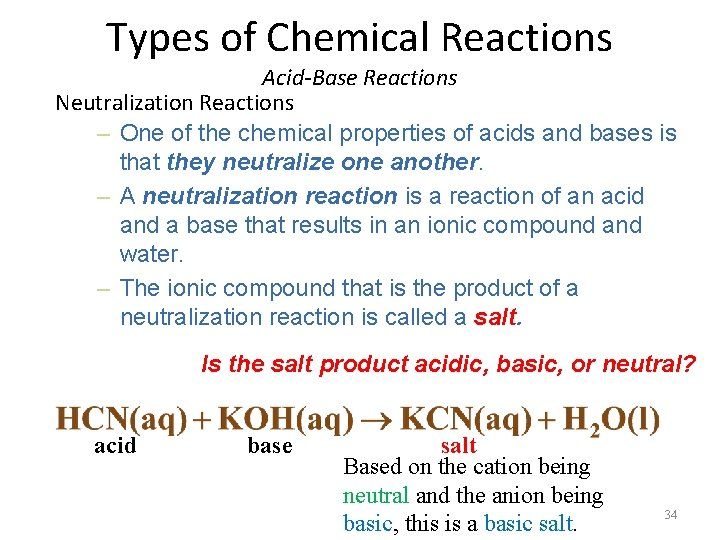Types of Chemical Reactions Acid-Base Reactions Neutralization Reactions – One of the chemical properties of acids and bases is that they neutralize one another. – A neutralization reaction is a reaction of an acid and a base that results in an ionic compound and water. – The ionic compound that is the product of a neutralization reaction is called a salt. Is the salt product acidic, basic, or neutral? acid base salt Based on the cation being neutral and the anion being basic, this is a basic salt. 34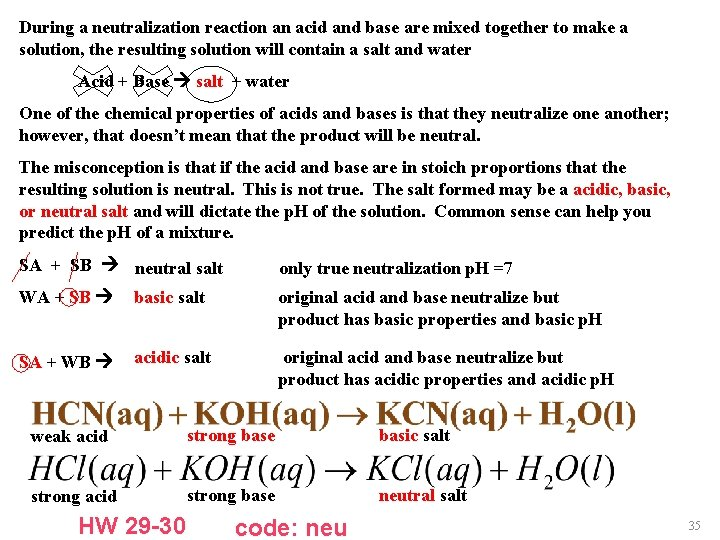During a neutralization reaction an acid and base are mixed together to make a solution, the resulting solution will contain a salt and water Acid + Base salt + water One of the chemical properties of acids and bases is that they neutralize one another; however, that doesn’t mean that the product will be neutral. The misconception is that if the acid and base are in stoich proportions that the resulting solution is neutral. This is not true. The salt formed may be a acidic, basic, or neutral salt and will dictate the p. H of the solution. Common sense can help you predict the p. H of a mixture. SA + SB neutral salt only true neutralization p. H =7 WA + SB basic salt original acid and base neutralize but product has basic properties and basic p. H SA + WB acidic salt original acid and base neutralize but product has acidic properties and acidic p. H weak acid strong base basic salt strong acid strong base neutral salt HW 29 -30 code: neu 35Types of Chemical Reactions Acid-Base Reactions with Gas Formation with certain salts – Carbonates react with acids to form CO 2, carbon dioxide gas. H 2 CO 3(aq) H 2 O(l) + CO 2(g) – Sulfites react with acids to form SO 2, sulfur dioxide gas. H SO (aq) H O + SO 2 3 2 (l) 2(g) – Sulfides react with acids to form H 2 S, hydrogen sulfide gas. 36Types of Chemical Reactions Acid-Base Reactions Other Acid-Base reactions Nonmetal oxides (or acid oxides or acid anhydrides) react with water to form acids SO 2 + H 2 O H 2 SO 3 Metal oxides (or basic oxides or basic anhydrides) react with water to form bases Na 2 O + H 2 O 2 Na. OH 37Types of Chemical Reactions Oxidation-Reduction Reactions (Redox rxn) – Oxidation-reduction reactions involve the transfer of electrons from one species to another. – Oxidation is defined as the loss of electrons (higher oxidation state). – Reduction is defined as the gain of electrons (lower oxidation state). – Oxidation and reduction always occur simultaneously. 38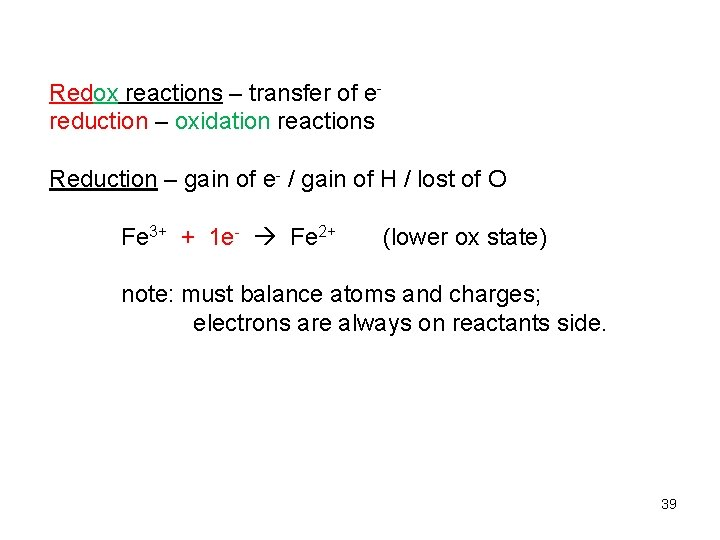Redox reactions – transfer of ereduction – oxidation reactions Reduction – gain of e- / gain of H / lost of O Fe 3+ + 1 e- Fe 2+ (lower ox state) note: must balance atoms and charges; electrons are always on reactants side. 39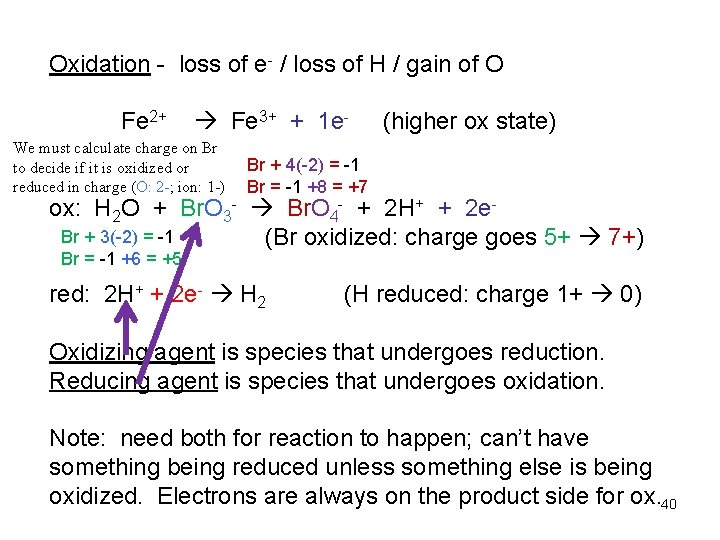Oxidation - loss of e- / loss of H / gain of O Fe 2+ Fe 3+ + 1 e- We must calculate charge on Br to decide if it is oxidized or reduced in charge (O: 2 -; ion: 1 -) (higher ox state) Br + 4(-2) = -1 Br = -1 +8 = +7 ox: H 2 O + Br. O 3 - Br. O 4 - + 2 H+ + 2 e. Br + 3(-2) = -1 (Br oxidized: charge goes 5+ 7+) Br = -1 +6 = +5 red: 2 H+ + 2 e- H 2 (H reduced: charge 1+ 0) Oxidizing agent is species that undergoes reduction. Reducing agent is species that undergoes oxidation. Note: need both for reaction to happen; can’t have something being reduced unless something else is being oxidized. Electrons are always on the product side for ox. 40Types of Chemical Reactions Oxidation-Reduction Reactions Copper prefers to be reduced while iron prefers to be oxidized when present together. Lets write a net ionic reaction for iron and copper (II) sulfate. soluble salt soluble salt total ionic: Fe(s) + Cu 2+(aq) + SO 42 -(aq) Fe 2+(aq) + SO 42 -(aq) + Cu(s) net ionic: Fe(s) + Cu 2+(aq) Fe 2+(aq) + Cu(s) 41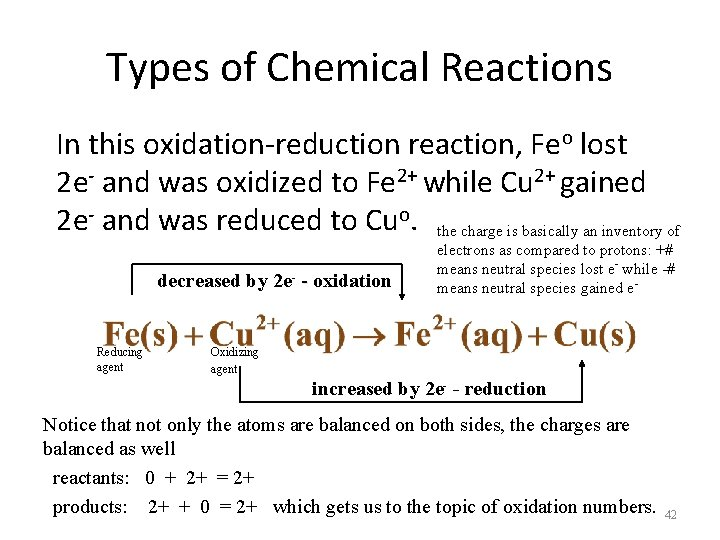Types of Chemical Reactions In this oxidation-reduction reaction, Feo lost 2 e- and was oxidized to Fe 2+ while Cu 2+ gained 2 e- and was reduced to Cuo. the charge is basically an inventory of decreased by 2 e- - oxidation - Reducing agent electrons as compared to protons: +# means neutral species lost e- while -# means neutral species gained e- Oxidizing agent increased by 2 e- - reduction Notice that not only the atoms are balanced on both sides, the charges are balanced as well reactants: 0 + 2+ = 2+ products: 2+ + 0 = 2+ which gets us to the topic of oxidation numbers. 42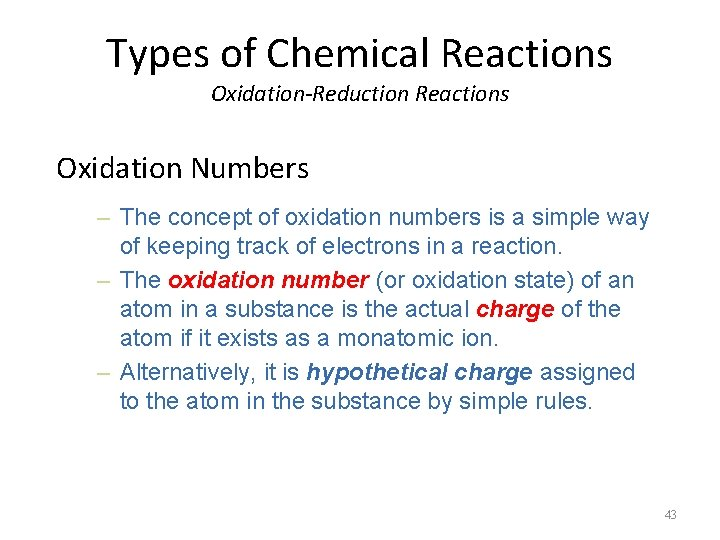Types of Chemical Reactions Oxidation-Reduction Reactions Oxidation Numbers – The concept of oxidation numbers is a simple way of keeping track of electrons in a reaction. – The oxidation number (or oxidation state) of an atom in a substance is the actual charge of the atom if it exists as a monatomic ion. – Alternatively, it is hypothetical charge assigned to the atom in the substance by simple rules. 43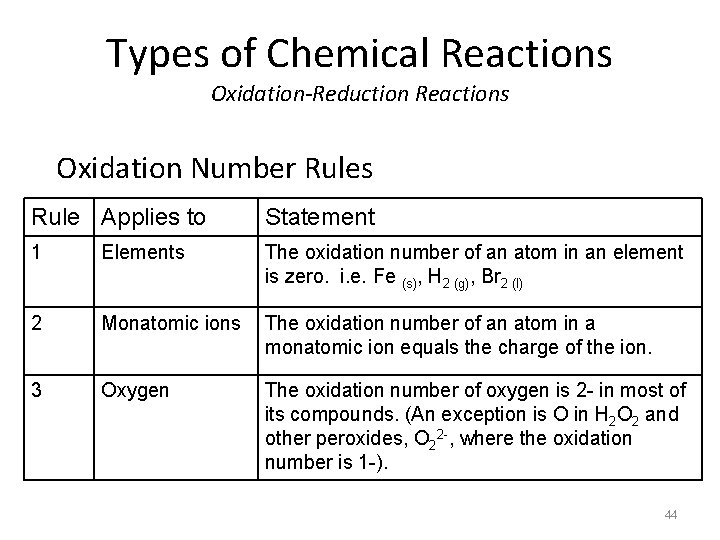Types of Chemical Reactions Oxidation-Reduction Reactions Oxidation Number Rules Rule Applies to Statement 1 Elements The oxidation number of an atom in an element is zero. i. e. Fe (s), H 2 (g), Br 2 (l) 2 Monatomic ions The oxidation number of an atom in a monatomic ion equals the charge of the ion. 3 Oxygen The oxidation number of oxygen is 2 - in most of its compounds. (An exception is O in H 2 O 2 and other peroxides, O 22 -, where the oxidation number is 1 -). 44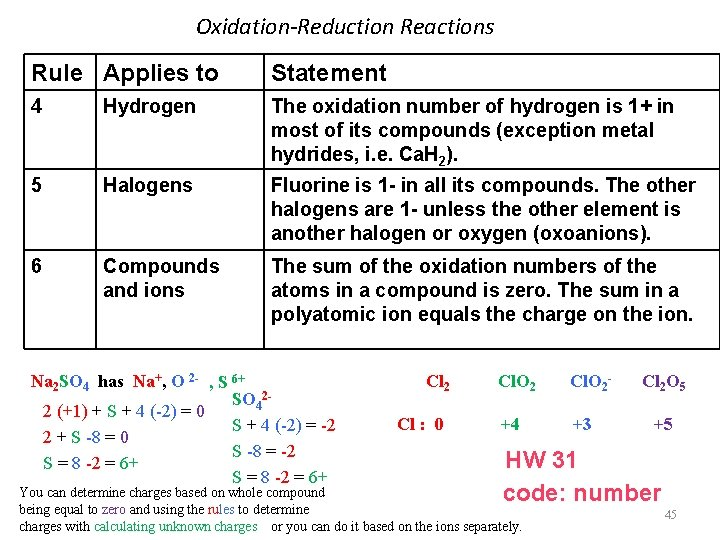Oxidation-Reduction Reactions Rule Applies to Statement 4 Hydrogen The oxidation number of hydrogen is 1+ in most of its compounds (exception metal hydrides, i. e. Ca. H 2). 5 Halogens Fluorine is 1 - in all its compounds. The other halogens are 1 - unless the other element is another halogen or oxygen (oxoanions). 6 Compounds and ions The sum of the oxidation numbers of the atoms in a compound is zero. The sum in a polyatomic ion equals the charge on the ion. Na 2 SO 4 has Na+, O 2 - , S 6+ SO 422 (+1) + S + 4 (-2) = 0 S + 4 (-2) = -2 2 + S -8 = 0 S -8 = -2 S = 8 -2 = 6+ Cl 2 Cl : 0 Cl. O 2 - +4 +3 Cl 2 O 5 +5 HW 31 code: number You can determine charges based on whole compound being equal to zero and using the rules to determine charges with calculating unknown charges or you can do it based on the ions separately. 45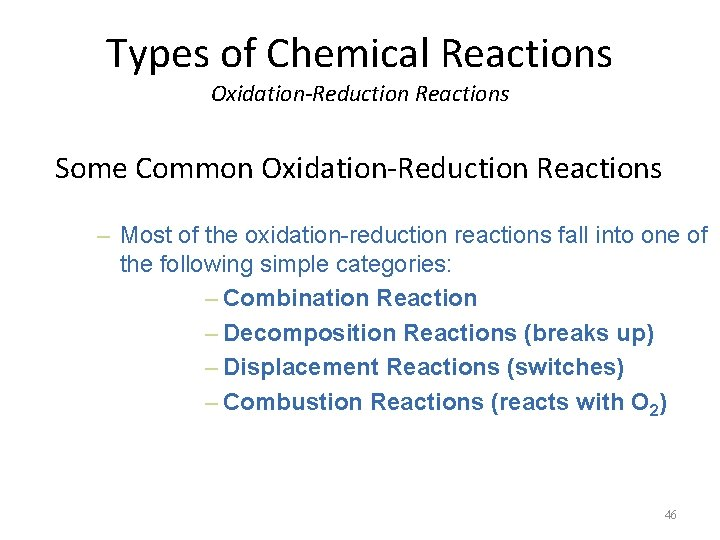Types of Chemical Reactions Oxidation-Reduction Reactions Some Common Oxidation-Reduction Reactions – Most of the oxidation-reduction reactions fall into one of the following simple categories: – Combination Reaction – Decomposition Reactions (breaks up) – Displacement Reactions (switches) – Combustion Reactions (reacts with O 2) 46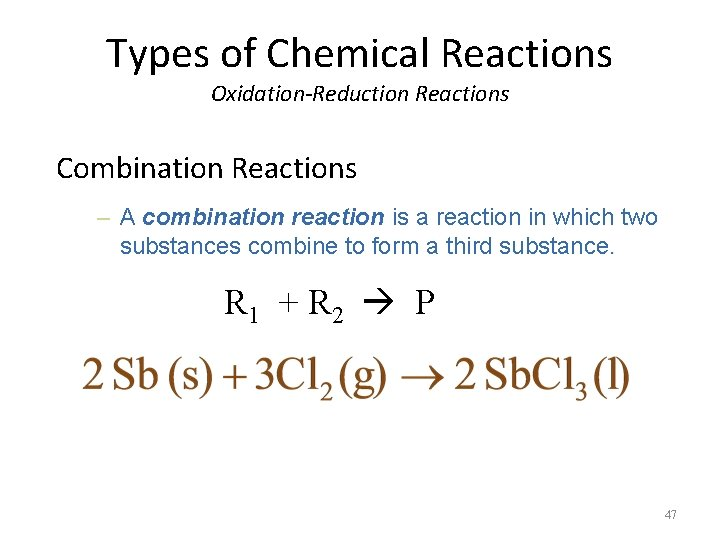Types of Chemical Reactions Oxidation-Reduction Reactions Combination Reactions – A combination reaction is a reaction in which two substances combine to form a third substance. R 1 + R 2 P 47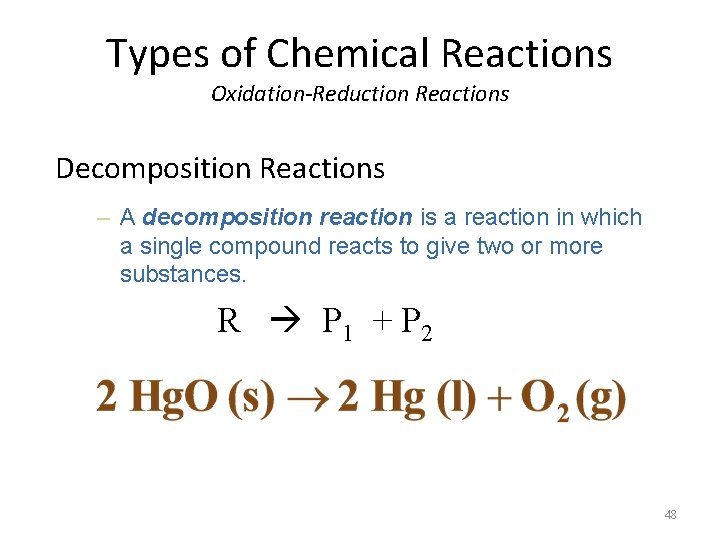Types of Chemical Reactions Oxidation-Reduction Reactions Decomposition Reactions – A decomposition reaction is a reaction in which a single compound reacts to give two or more substances. R P 1 + P 2 48Types of Chemical Reactions Oxidation-Reduction Reactions Displacement Reactions – A displacement reaction (also called a singlereplacement reaction) is a reaction in which an element reacts with a compound, displacing an element from it. 49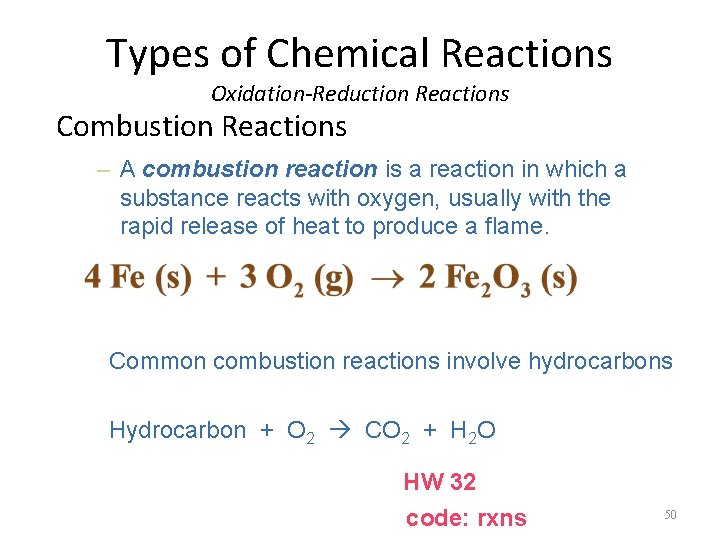Types of Chemical Reactions Oxidation-Reduction Reactions Combustion Reactions – A combustion reaction is a reaction in which a substance reacts with oxygen, usually with the rapid release of heat to produce a flame. Common combustion reactions involve hydrocarbons Hydrocarbon + O 2 CO 2 + H 2 O HW 32 code: rxns 50Types of Chemical Reactions Oxidation-Reduction Reactions How do we balance Oxidation-Reduction Reactions? Look again at the net reaction of iron with copper(II) sulfate. We can write this reaction in terms of two halfreactions. 51Types of Chemical Reactions Oxidation-Reduction Reactions A half-reaction is one of the two parts of an oxidation-reduction reaction. One involves the loss of electrons (oxidation) and the other involves the gain of electrons (reduction). To write a balanced redox reaction, you must write the half-reactions, balance the atoms, balance the charges, and finally balance the number of electrons transferred. oxidation half-reaction reduction half-reaction Notice: atoms and charges are balanced. + 2 e- + 2 e _______________ 52Balancing Redox Equations - Must know charges (oxidation numbers) of species including polyatomic ions - Must know strong/weak acids and bases - Must know the solubility rules 53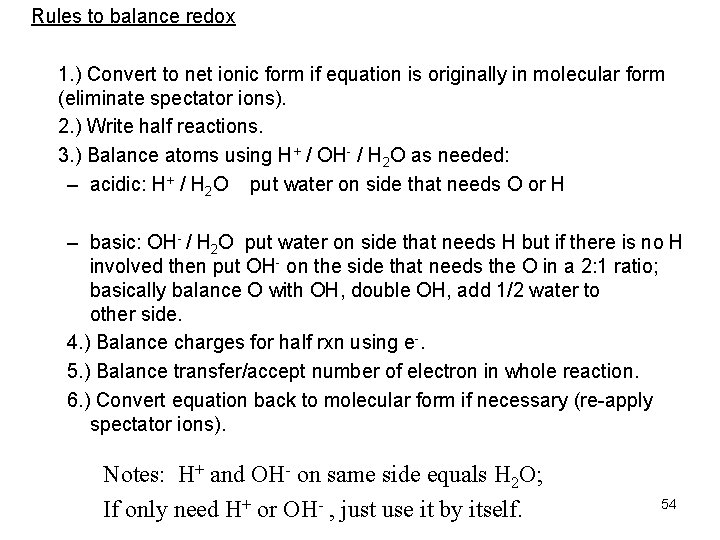Rules to balance redox 1. ) Convert to net ionic form if equation is originally in molecular form (eliminate spectator ions). 2. ) Write half reactions. 3. ) Balance atoms using H+ / OH- / H 2 O as needed: – acidic: H+ / H 2 O put water on side that needs O or H – basic: OH- / H 2 O put water on side that needs H but if there is no H involved then put OH- on the side that needs the O in a 2: 1 ratio; basically balance O with OH, double OH, add 1/2 water to other side. 4. ) Balance charges for half rxn using e-. 5. ) Balance transfer/accept number of electron in whole reaction. 6. ) Convert equation back to molecular form if necessary (re-apply spectator ions). Notes: H+ and OH- on same side equals H 2 O; If only need H+ or OH- , just use it by itself. 54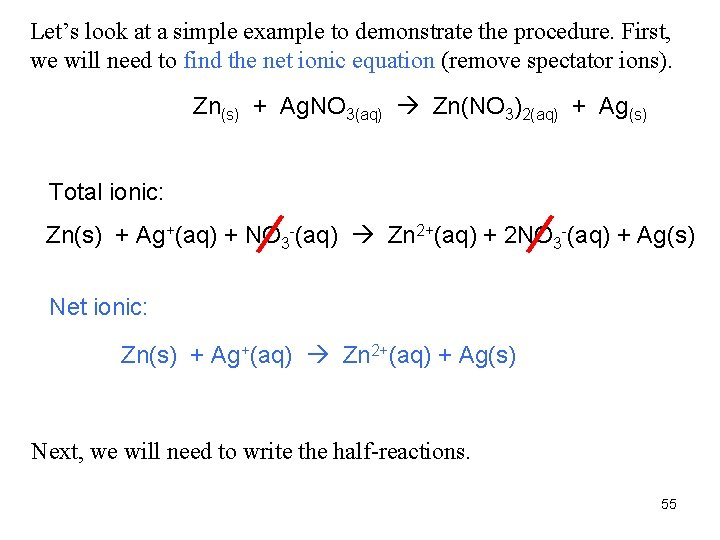Let’s look at a simple example to demonstrate the procedure. First, we will need to find the net ionic equation (remove spectator ions). Zn(s) + Ag. NO 3(aq) Zn(NO 3)2(aq) + Ag(s) Total ionic: Zn(s) + Ag+(aq) + NO 3 -(aq) Zn 2+(aq) + 2 NO 3 -(aq) + Ag(s) Net ionic: Zn(s) + Ag+(aq) Zn 2+(aq) + Ag(s) Next, we will need to write the half-reactions. 55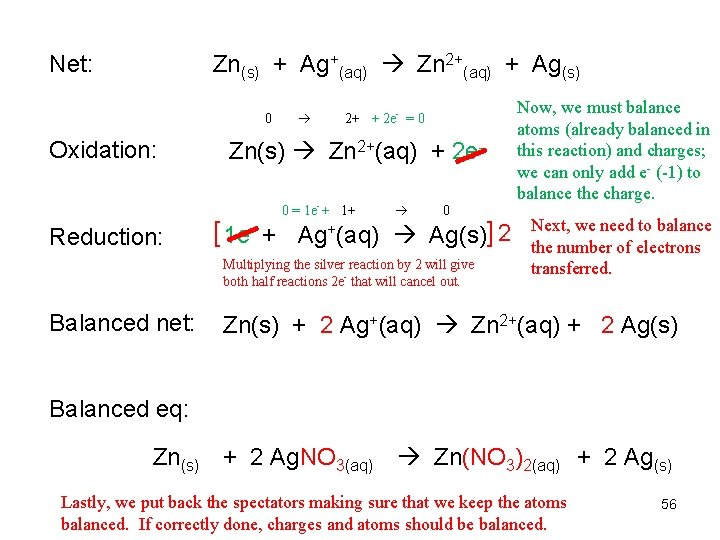Net: Zn(s) + Ag+(aq) Zn 2+(aq) + Ag(s) 0 2+ + 2 e- = 0 Oxidation: Zn(s) Zn 2+(aq) + 2 e 0 = 1 e- + 1+ 0 Reduction: [ 1 e- + Ag+(aq) Ag(s)] 2 Multiplying the silver reaction by 2 will give both half reactions 2 e- that will cancel out. Balanced net: Now, we must balance atoms (already balanced in this reaction) and charges; we can only add e- (-1) to balance the charge. Next, we need to balance the number of electrons transferred. Zn(s) + 2 Ag+(aq) Zn 2+(aq) + 2 Ag(s) Balanced eq: Zn(s) + 2 Ag. NO 3(aq) Zn(NO 3)2(aq) + 2 Ag(s) Lastly, we put back the spectators making sure that we keep the atoms balanced. If correctly done, charges and atoms should be balanced. 56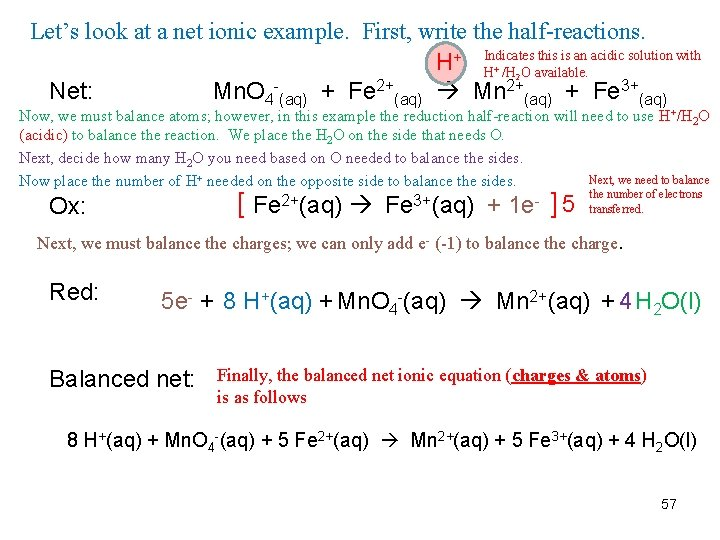Let’s look at a net ionic example. First, write the half-reactions. H+ Indicates this is an acidic solution with H /H O available. 2+ Net: Mn. O 4 (aq) + Fe (aq) Mn 2+(aq) + Fe 3+(aq) + 2 Now, we must balance atoms; however, in this example the reduction half-reaction will need to use H+/H 2 O (acidic) to balance the reaction. We place the H 2 O on the side that needs O. Next, decide how many H 2 O you need based on O needed to balance the sides. Next, we need to balance Now place the number of H+ needed on the opposite side to balance the sides. the number of electrons 2+ 3+ 5 transferred. Fe (aq) + 1 e [ Ox: ] Next, we must balance the charges; we can only add e- (-1) to balance the charge. Red: 5 e- + 8 H+(aq) + Mn. O 4 -(aq) Mn 2+(aq) + 4 H 2 O(l) Balanced net: Finally, the balanced net ionic equation (charges & atoms) is as follows 8 H+(aq) + Mn. O 4 -(aq) + 5 Fe 2+(aq) Mn 2+(aq) + 5 Fe 3+(aq) + 4 H 2 O(l) 57Let’s look at a molecular equation example. First, we need the net eq. KMn. O 4(aq) + Na. NO 2(aq) + HCl(aq) Na. NO 3(aq) + Mn. Cl 2(aq) + KCl(aq) + H 2 O(l) Net: Mn. O 4 -(aq) + NO 2 -(aq) + H+(aq) NO 3 -(aq) + Mn 2+(aq) + H 2 O(l) Noticed that after we removed the spectators, KCl is not part of the net. We must remember to put it back at the end. H 2 O(l) + NO 2 -(aq) NO 3 -(aq) + 2 H+(aq) + 2 e- ] 5 Ox: [ Red: [ 5 e- + 8 H+(aq) + Mn. O 4 -(aq) Mn 2+(aq) + 4 H 2 O(l) ] 2 Balanced net: Equation can be reduced since it has common species on both sides. 2 Mn. O 4 -(aq) + 5 NO 2 -(aq) + 16 H+(aq) + 5 H 2 O(l) 2 Mn 2+(aq) + 8 H 2 O(l) + 5 NO 3 -(aq) +10 H+(aq) 2 Mn. O 4 -(aq) + 5 NO 2 -(aq) + 6 H+(aq) 2 Mn 2+(aq) + 3 H 2 O(l) + 5 NO 3 -(aq) Balanced eq: 2 KMn. O 4(aq) + 5 Na. NO 2(aq) + 6 HCl(aq) 2 Mn. Cl 2(aq)+ 3 H 2 O(l)+ 5 Na. NO 3(aq) + 2 KCl Lastly, we put back the spectators making sure that we keep the atoms balanced. 58 Note: we must add back KCl and balanced K and Cl for the entire reaction can be balanced.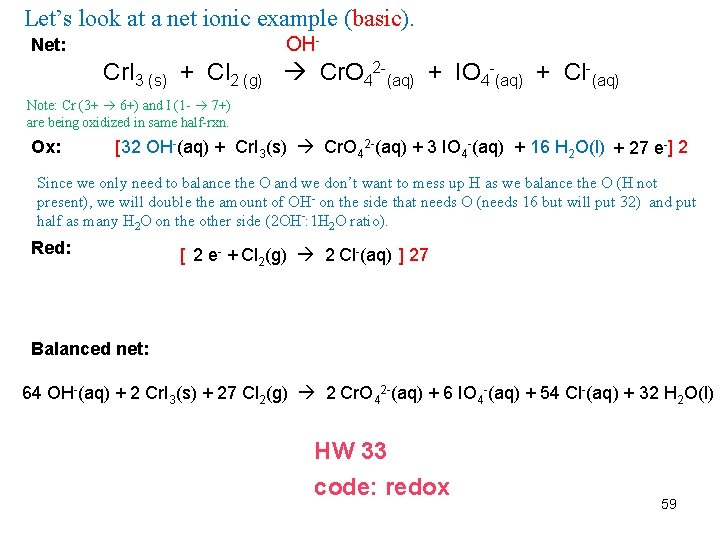Let’s look at a net ionic example (basic). OH- Net: Cr. I 3 (s) + Cl 2 (g) Cr. O 42 -(aq) + IO 4 -(aq) + Cl-(aq) Note: Cr (3+ 6+) and I (1 - 7+) are being oxidized in same half-rxn. Ox: [ 32 OH-(aq) + Cr. I 3(s) Cr. O 42 -(aq) + 3 IO 4 -(aq) + 16 H 2 O(l) + 27 e- ] 2 Since we only need to balance the O and we don’t want to mess up H as we balance the O (H not present), we will double the amount of OH- on the side that needs O (needs 16 but will put 32) and put half as many H 2 O on the other side (2 OH-: 1 H 2 O ratio). Red: [ 2 e- + Cl 2(g) 2 Cl-(aq) ] 27 Balanced net: 64 OH-(aq) + 2 Cr. I 3(s) + 27 Cl 2(g) 2 Cr. O 42 -(aq) + 6 IO 4 -(aq) + 54 Cl-(aq) + 32 H 2 O(l) HW 33 code: redox 59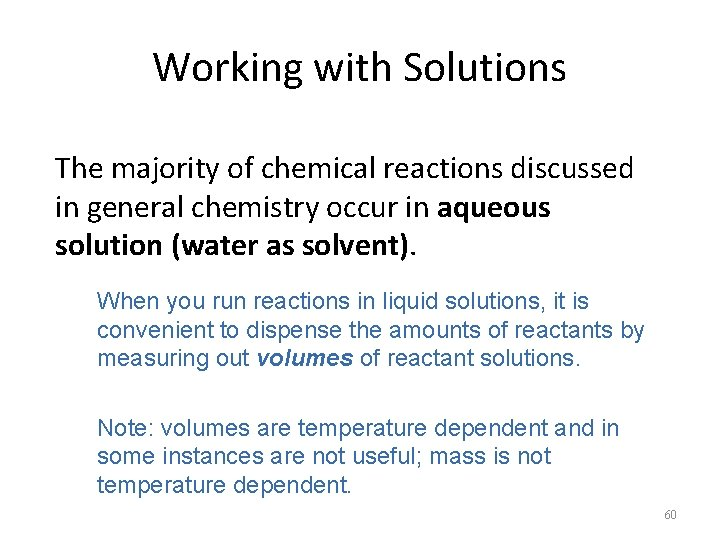Working with Solutions The majority of chemical reactions discussed in general chemistry occur in aqueous solution (water as solvent). When you run reactions in liquid solutions, it is convenient to dispense the amounts of reactants by measuring out volumes of reactant solutions. Note: volumes are temperature dependent and in some instances are not useful; mass is not temperature dependent. 60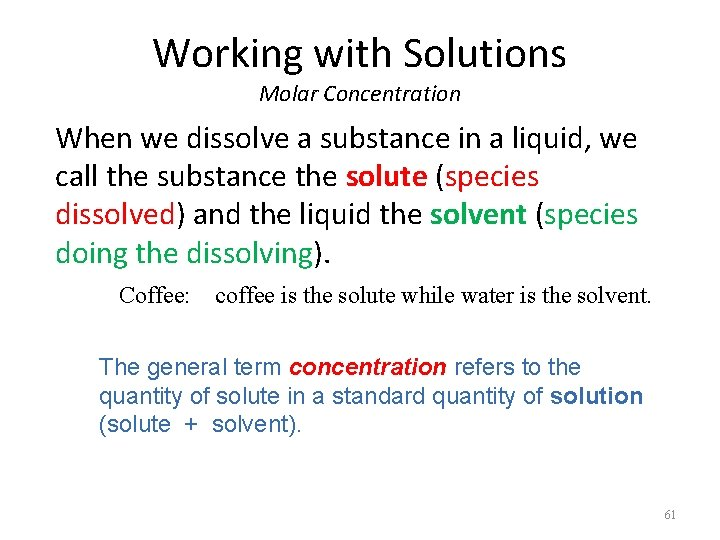Working with Solutions Molar Concentration When we dissolve a substance in a liquid, we call the substance the solute (species dissolved) and the liquid the solvent (species doing the dissolving). Coffee: coffee is the solute while water is the solvent. The general term concentration refers to the quantity of solute in a standard quantity of solution (solute + solvent). 61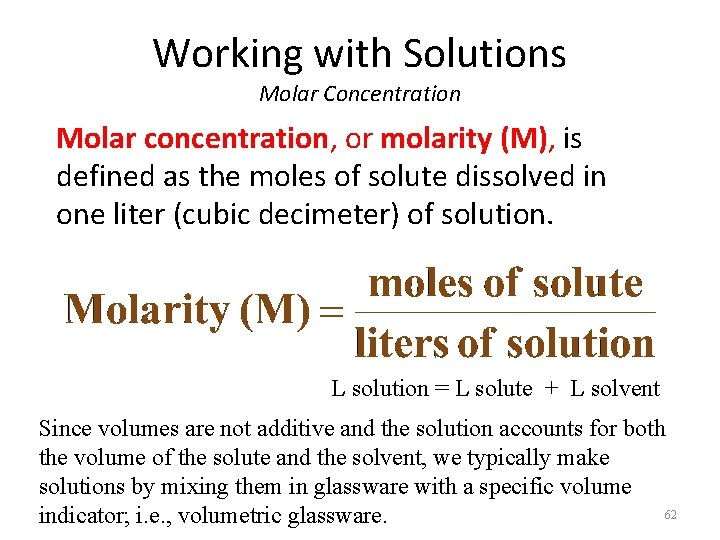Working with Solutions Molar Concentration Molar concentration, or molarity (M), is defined as the moles of solute dissolved in one liter (cubic decimeter) of solution. L solution = L solute + L solvent Since volumes are not additive and the solution accounts for both the volume of the solute and the solvent, we typically make solutions by mixing them in glassware with a specific volume 62 indicator; i. e. , volumetric glassware.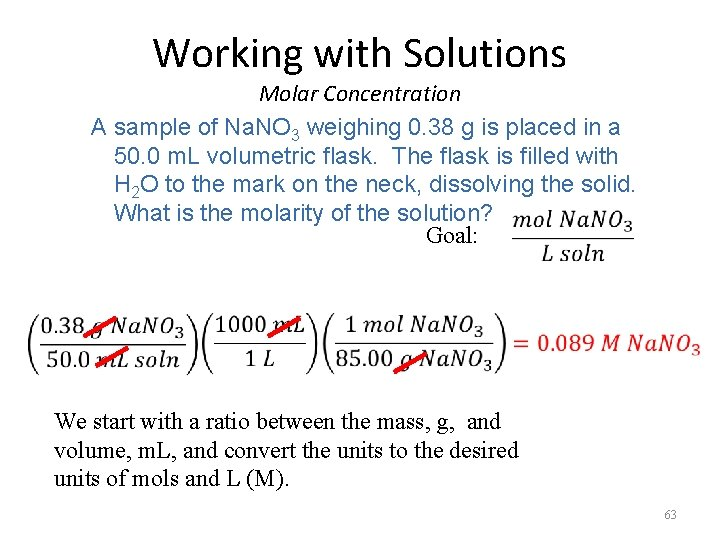Working with Solutions Molar Concentration A sample of Na. NO 3 weighing 0. 38 g is placed in a 50. 0 m. L volumetric flask. The flask is filled with H 2 O to the mark on the neck, dissolving the solid. What is the molarity of the solution? Goal: We start with a ratio between the mass, g, and volume, m. L, and convert the units to the desired units of mols and L (M). 63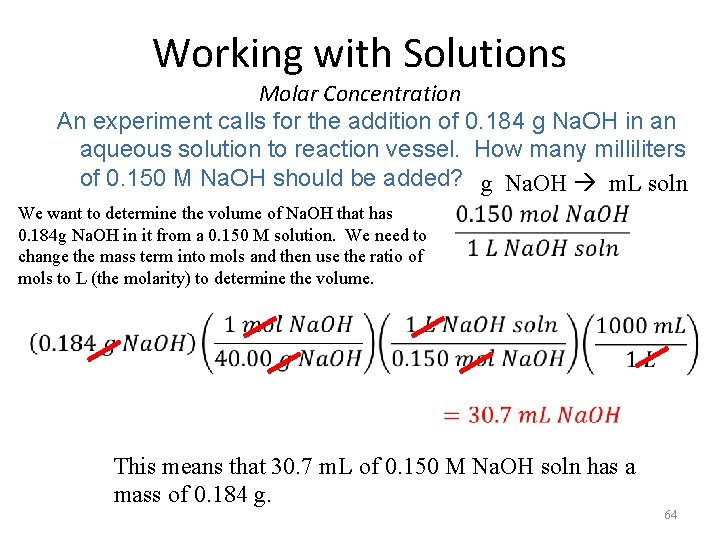Working with Solutions Molar Concentration An experiment calls for the addition of 0. 184 g Na. OH in an aqueous solution to reaction vessel. How many milliliters of 0. 150 M Na. OH should be added? g Na. OH m. L soln We want to determine the volume of Na. OH that has 0. 184 g Na. OH in it from a 0. 150 M solution. We need to change the mass term into mols and then use the ratio of mols to L (the molarity) to determine the volume. This means that 30. 7 m. L of 0. 150 M Na. OH soln has a mass of 0. 184 g. 64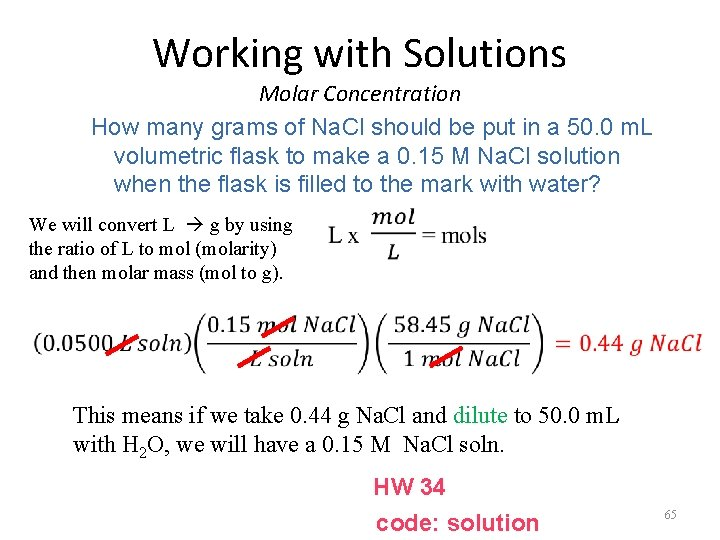Working with Solutions Molar Concentration How many grams of Na. Cl should be put in a 50. 0 m. L volumetric flask to make a 0. 15 M Na. Cl solution when the flask is filled to the mark with water? We will convert L g by using the ratio of L to mol (molarity) and then molar mass (mol to g). This means if we take 0. 44 g Na. Cl and dilute to 50. 0 m. L with H 2 O, we will have a 0. 15 M Na. Cl soln. HW 34 code: solution 65Working with Solutions Molar Concentration The molarity of a solution and its volume are inversely proportional. Therefore, adding water makes the solution less concentrated. This inverse relationship takes the form of: This equation can be used for any concentration term, C, where c – 66 concentrated and d – diluted. Only use for dilution calculations.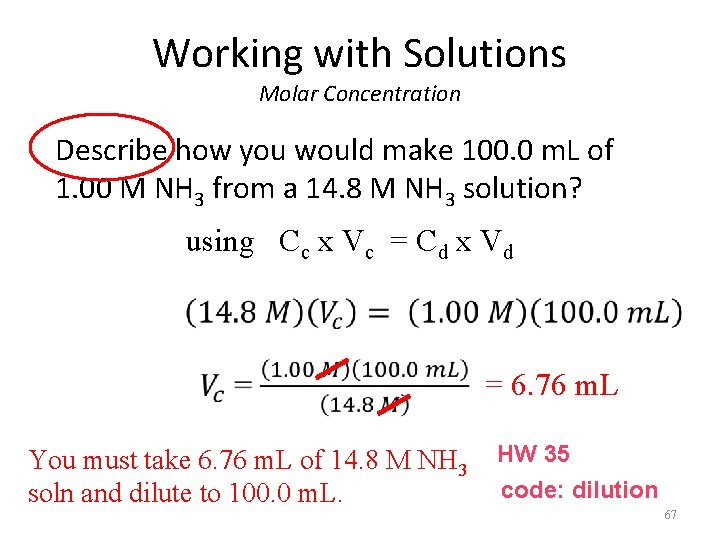Working with Solutions Molar Concentration Describe how you would make 100. 0 m. L of 1. 00 M NH 3 from a 14. 8 M NH 3 solution? using Cc x Vc = Cd x Vd = 6. 76 m. L You must take 6. 76 m. L of 14. 8 M NH 3 HW 35 code: dilution soln and dilute to 100. 0 m. L. 67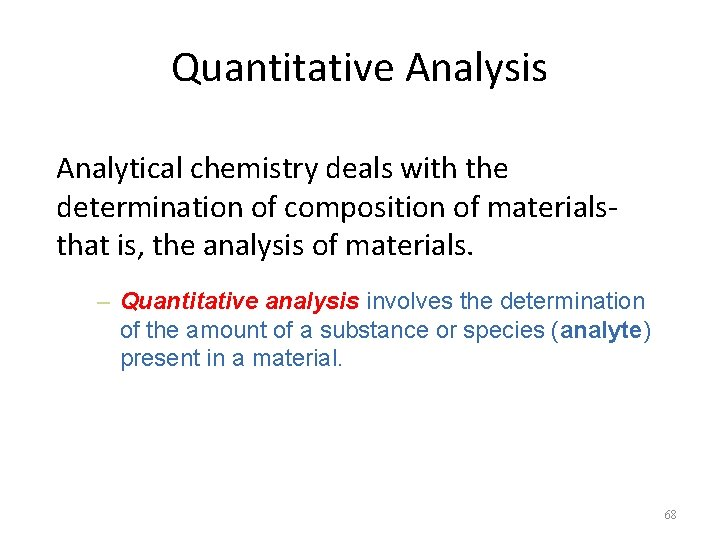Quantitative Analysis Analytical chemistry deals with the determination of composition of materialsthat is, the analysis of materials. – Quantitative analysis involves the determination of the amount of a substance or species (analyte) present in a material. 68Quantitative Analysis Gravimetric analysis is a type of quantitative analysis in which the amount of a species in a material is determined by converting the species into a product that can be isolated and weighed. – Precipitation reactions are often used in gravimetric analysis. – The precipitate from these reactions is then filtered, dried, and weighed. – If the end product is the analyte, you can determine the quantity directly or if the end product is not the analyte, mol-to-mol ratios can be used to indirectly 69 determine the quantity of analyte.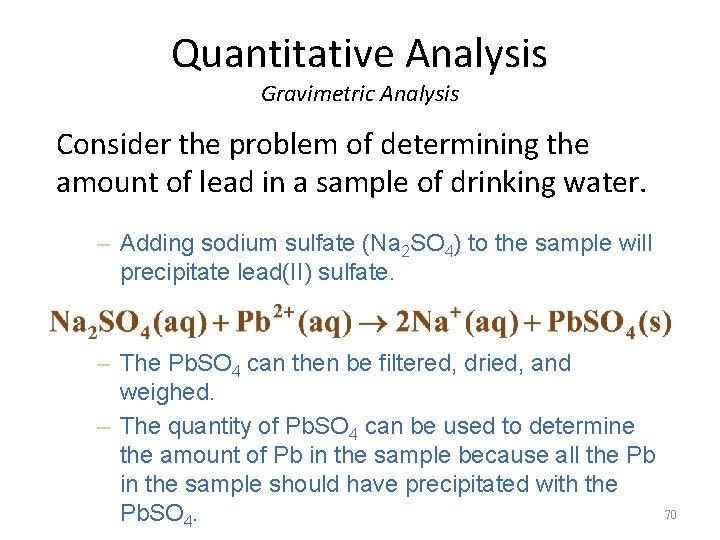Quantitative Analysis Gravimetric Analysis Consider the problem of determining the amount of lead in a sample of drinking water. – Adding sodium sulfate (Na 2 SO 4) to the sample will precipitate lead(II) sulfate. – The Pb. SO 4 can then be filtered, dried, and weighed. – The quantity of Pb. SO 4 can be used to determine the amount of Pb in the sample because all the Pb in the sample should have precipitated with the Pb. SO 4. 70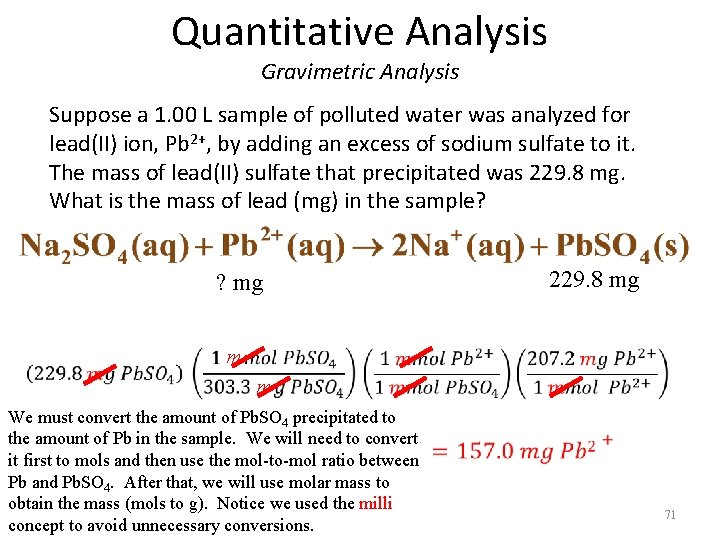Quantitative Analysis Gravimetric Analysis Suppose a 1. 00 L sample of polluted water was analyzed for lead(II) ion, Pb 2+, by adding an excess of sodium sulfate to it. The mass of lead(II) sulfate that precipitated was 229. 8 mg. What is the mass of lead (mg) in the sample? 229. 8 mg ? mg m m We must convert the amount of Pb. SO 4 precipitated to the amount of Pb in the sample. We will need to convert it first to mols and then use the mol-to-mol ratio between Pb and Pb. SO 4. After that, we will use molar mass to obtain the mass (mols to g). Notice we used the milli concept to avoid unnecessary conversions. 71Quantitative Analysis Volumetric Analysis An important method for determining the amount of a particular substance is based on measuring the volume of the reactant solution. – Titration is a procedure for determining the amount of unknown substance A by adding a carefully measured volume of a solution with known concentration of B until the reaction of A and B is just complete (equivalence point: #mols of titrant = #mols analyte by stoich). – Volumetric analysis is a method of analysis based on titration. 72Quantitative Analysis Volumetric Analysis Consider the reaction of sulfuric acid, H 2 SO 4, with sodium hydroxide, Na. OH (note: must write a balanced equation to determine mol-tomol ratios): Suppose a beaker contains 35. 0 m. L of 0. 175 M H 2 SO 4. How many milliliters of 0. 250 M Na. OH must be added to completely react with the sulfuric acid? H 2 SO 4 (aq) + 2 Na. OH (aq) Na 2 SO 4 (aq) + 2 H 2 O (l) 35. 0 m. L 0. 175 M 0. 250 M ? m. L Note: volume times M gives us mols of H 2 SO 4 used. Next, we can do a mol-to-mol ratio to Na. OH and then determine volume from molarity (mol to L ratio). We will take advantage of the milli prefix once again. 73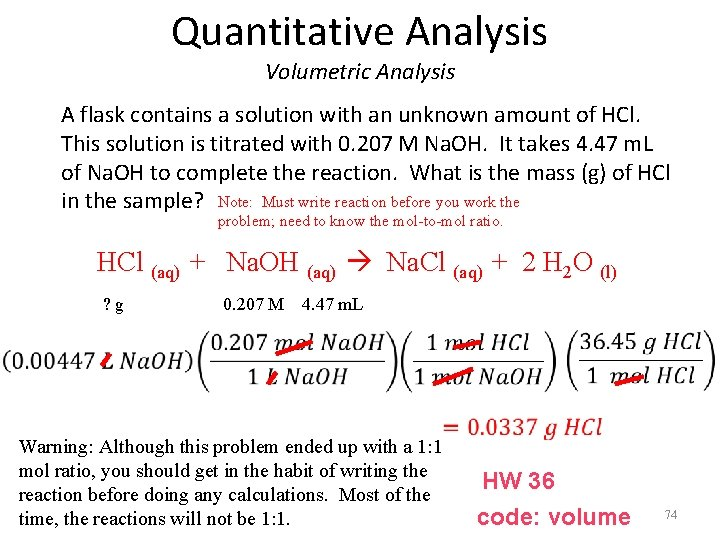Quantitative Analysis Volumetric Analysis A flask contains a solution with an unknown amount of HCl. This solution is titrated with 0. 207 M Na. OH. It takes 4. 47 m. L of Na. OH to complete the reaction. What is the mass (g) of HCl in the sample? Note: Must write reaction before you work the problem; need to know the mol-to-mol ratio. HCl (aq) + Na. OH (aq) Na. Cl (aq) + 2 H 2 O (l) ? g 0. 207 M 4. 47 m. L Warning: Although this problem ended up with a 1: 1 mol ratio, you should get in the habit of writing the reaction before doing any calculations. Most of the time, the reactions will not be 1: 1. HW 36 code: volume 74